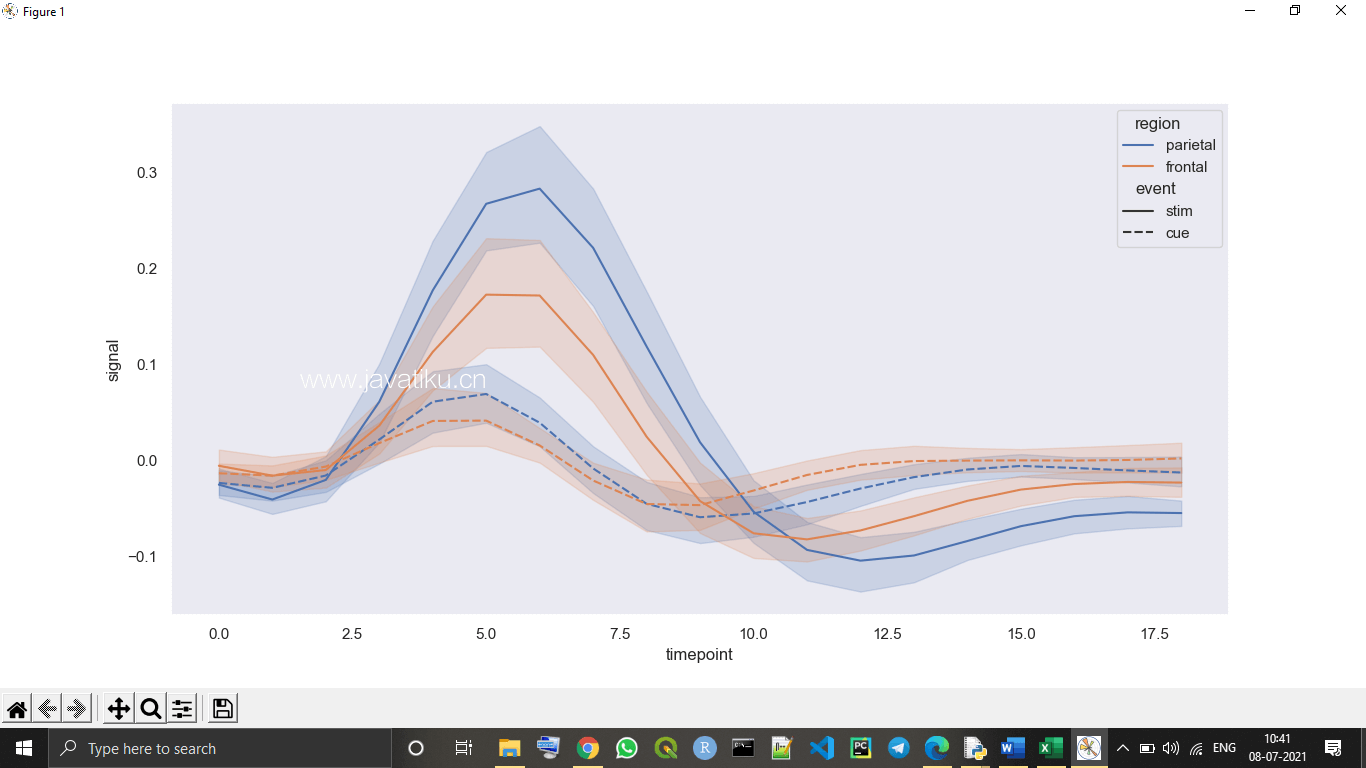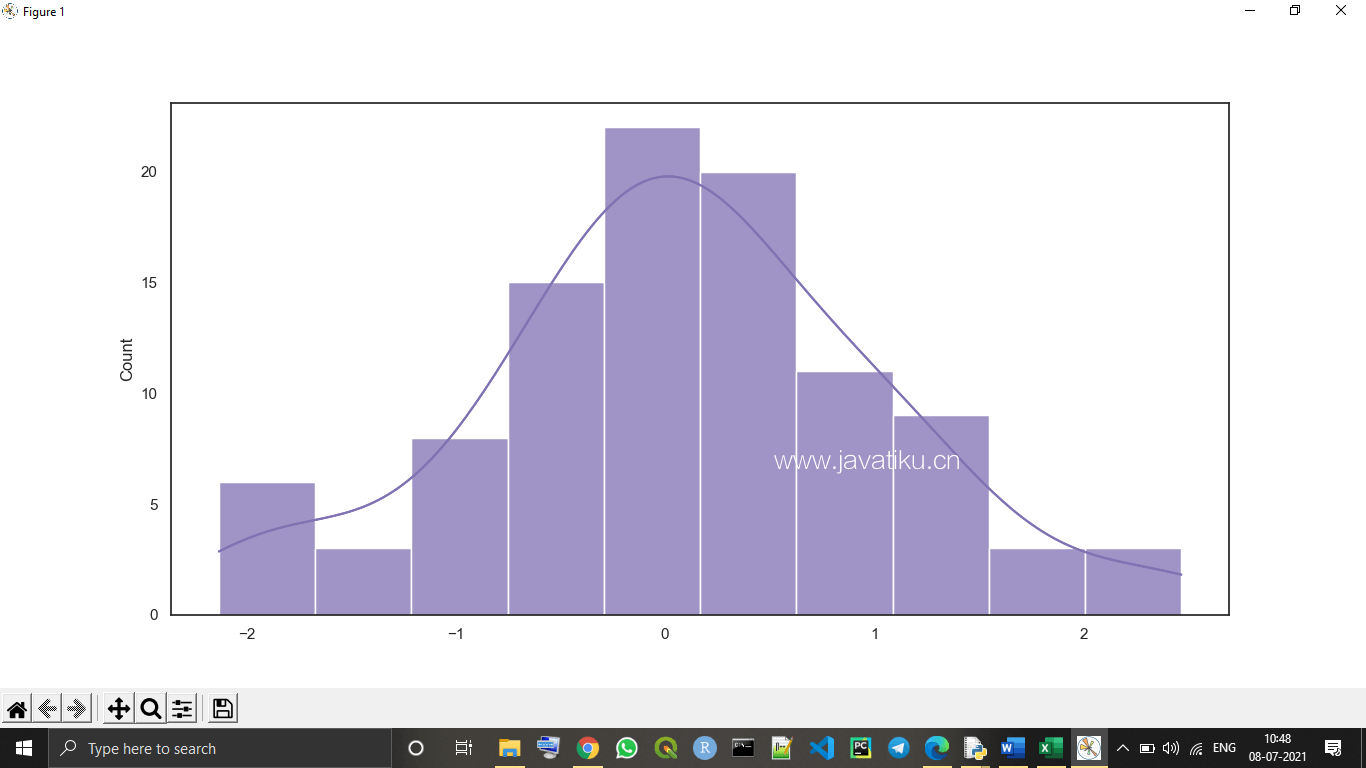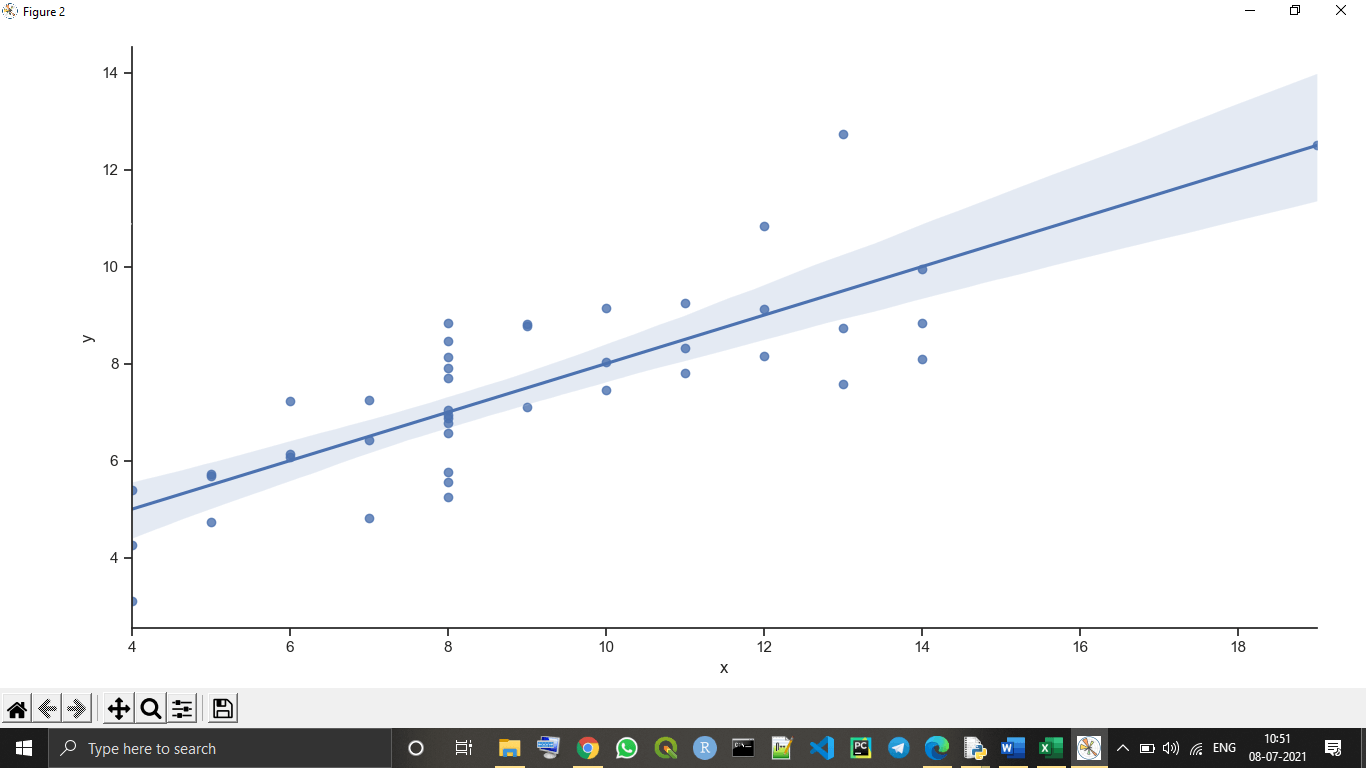# Python教程-Python seaborn库Seaborn是Python中用于图形统计绘图的一个惊人库之一。Seaborn提供了许多配色方案和默认的美观样式，以使Python中许多统计图的创建更具吸引力。

## Python Seaborn库的目标

Seaborn库旨在使对数据的中心部分进行更吸引人的可视化，以便更好地理解和探索数据。它建立在matplotlib库的核心之上，还提供了面向数据集的API。

Seaborn还与Pandas数据结构密切集成，借助它，我们可以轻松地在给定变量的各种不同视觉表示之间切换，以更好地理解提供的数据集。

## Python Seaborn库中的绘图类别

• 分布图： 用于检查两种类型的分布，即单变量和双变量分布。
• 关系图： 用于理解两个给定变量之间的关系。
• 回归图： Seaborn库中的回归图主要用于添加额外的视觉指南，有助于在探索性数据分析过程中强调数据集的模式。
• 分类图： 用于处理变量的各个类别以及如何可视化它们。
• 多图网格： 多图网格也是一种类型的图，一种有用的方法是在单个数据集的不同子集上绘制相同图的多个实例。
• 矩阵图： 矩阵图是一种散点图数组。

## 安装Python的seaborn库

``pip install seaborn``

seaborn库的必需依赖或先决条件：

• 安装了最新版本（3.6+）的Python。
• 必须安装了Numpy，版本为1.13.3或更高版本。
• 必须安装了SciPy，版本为1.0.1或更高版本。
• 必须安装了pandas库，版本为0.22.0或更高版本。
• 必须安装了statsmodel库，版本为0.8.0或更高版本。
• 必须安装了matplotlib，版本为2.1.2或更高版本。

## 使用seaborn库绘制图表

### 1. 折线图：

seaborn折线图是seaborn库中存在的最基本的绘图之一。我们主要使用seaborn折线图以一种连续的方式可视化给定的数据，即与时间相关。

``````# Importing seaborn library in program
import seaborn as sns
# Importing mataplotlib library to show graph in output
import matplotlib.pyplot as plt
# Setting style with set() function
sns.set(style="dark")
# Using dataset() function to declare data type
# Plotting various responses for different\
# Regions and events
sns.lineplot(x="timepoint",
y="signal",
hue="region",
style="event",
data=FMR) # using lineplot() function to create line plot
plt.show() # using show() function  ``````### 2. 分布图：

``````# importing numpy as np library module
import numpy as np
# Importing seaborn library in program
import seaborn as sns
# Importing mataplotlib library to show graph in output
import matplotlib.pyplot as plt
# Selecting style for boxplot with set() function
sns.set(style="white")
# Generate a random univariate type distribution
ru = np.random.RandomState(10)
d = ru.normal(size=100)
# Plotting a simple histogram with kdeplot variation
sns.histplot(d, kde=True, color="m")
plot = sns.histplot(d, kde=True, color="m")
print(plot)
plt.show() # using show() function  ``````### 3. Lmplot：

Lmplot是seaborn库中的另一个基本图之一。Lmplot显示代表线性回归模型的线与给定二维（2-D）空间上的数据点。在这个2-D空间中，我们可以将x和y变量分别设置为垂直和水平标签。

``````# Importing seaborn library in program
import seaborn as sns
# Importing matplotlib library to show graph in output
import matplotlib.pyplot as plt
# Using set() function to set style
sns.set(style="ticks")
# Using dataset() function
``<seaborn.axisgrid.FacetGrid object at 0x000002182DC89070>``